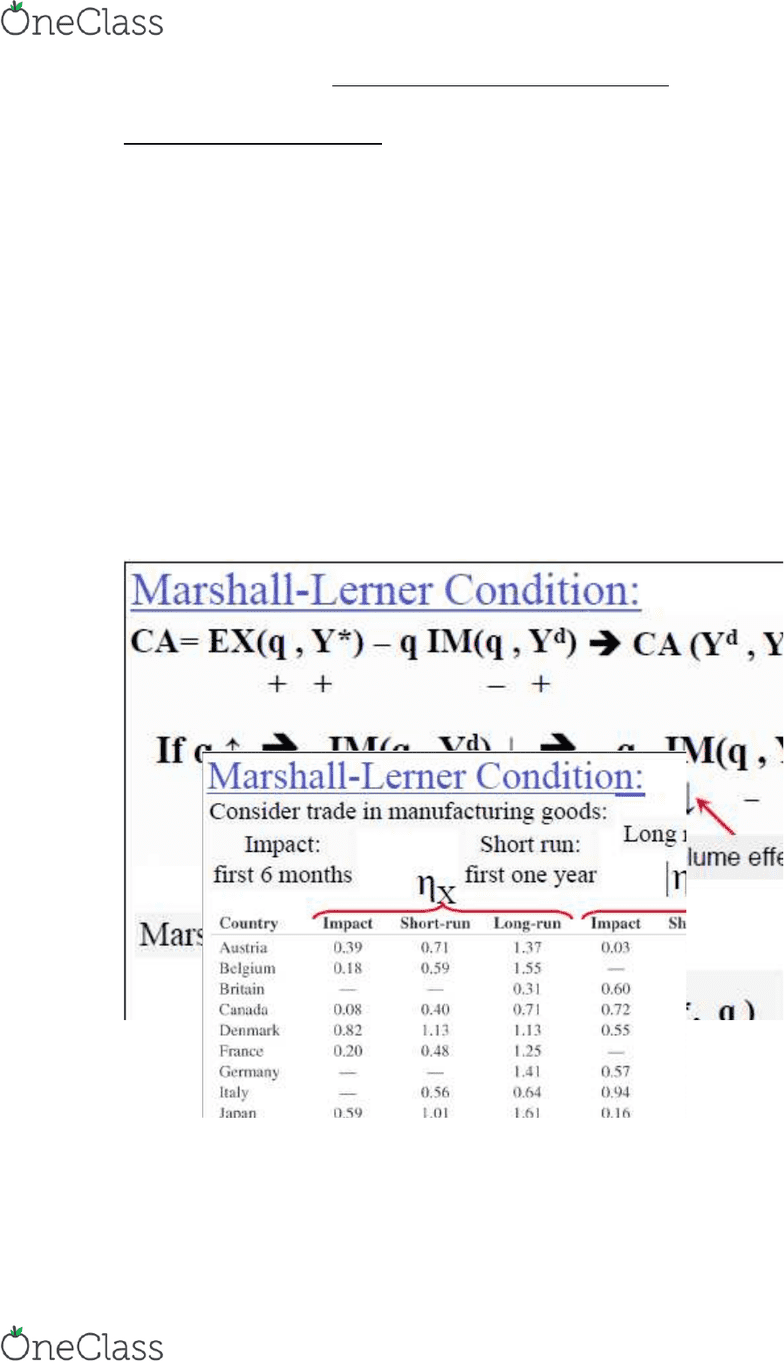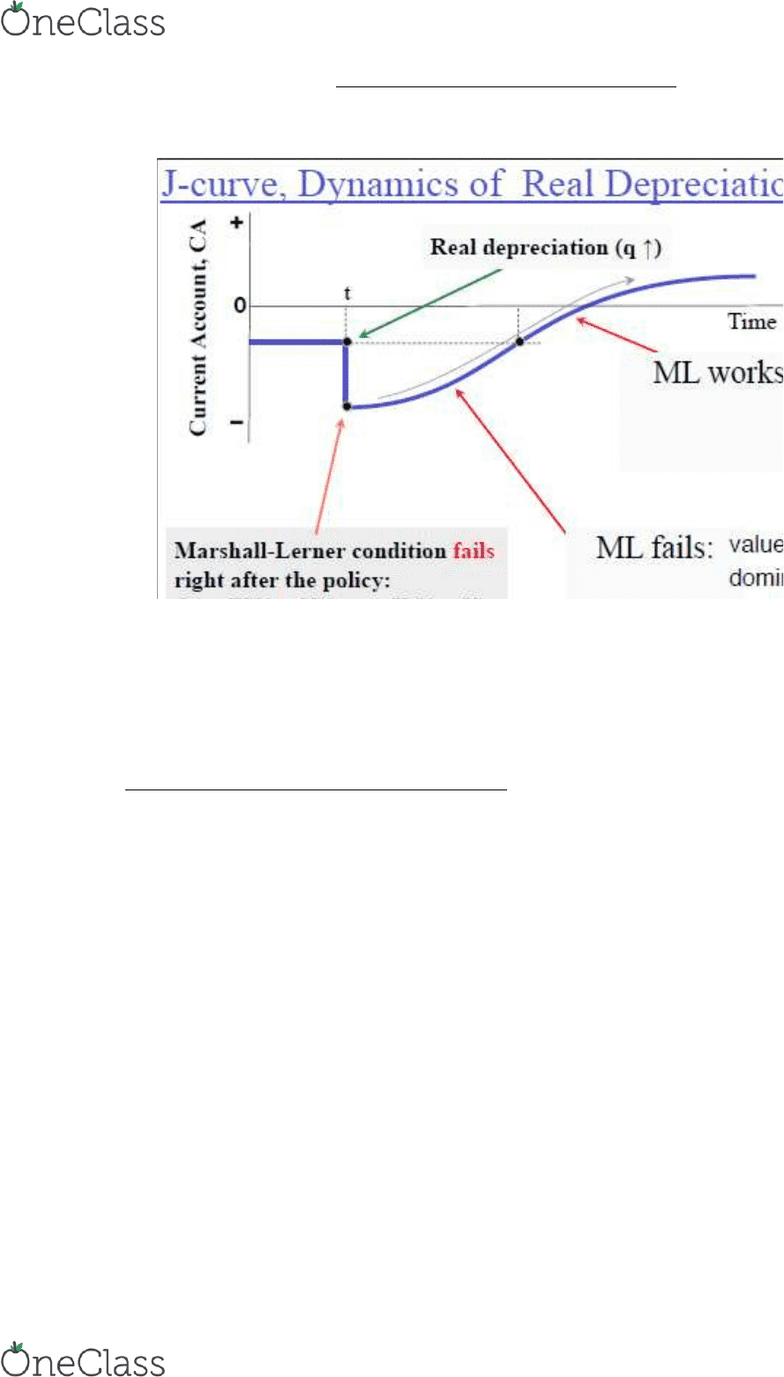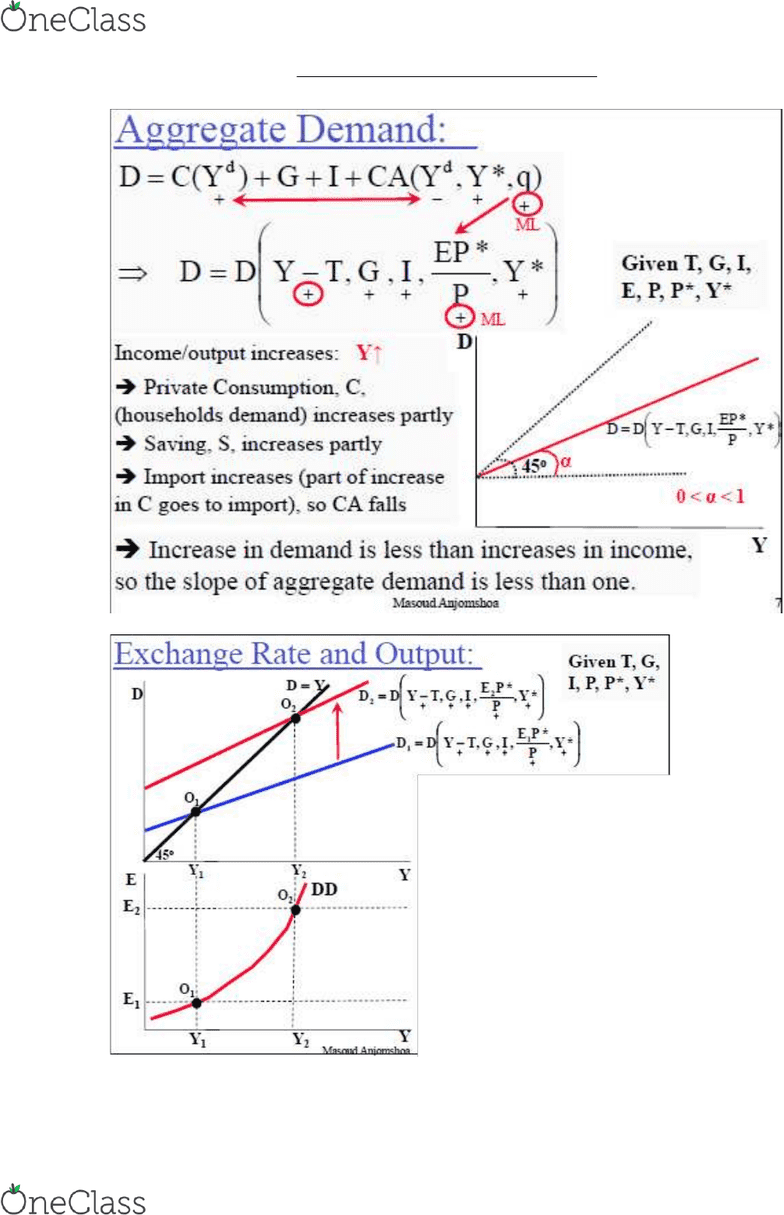Class Notes (1,100,000)
CA (650,000)
UTSG (50,000)
ECO (2,000)
Lecture 22

# ECO230Y1 Lecture Notes - Lecture 22: Capital Outflow, Price Discrimination, Interest Rate

Department
Economics
Course Code
ECO230Y1
Professor
Masoud Anjomshoa
Lecture
22

This preview shows pages 1-3. to view the full 11 pages of the document.Output and Exchange Rate
Chapter 17
Output and Exchange Rate:
Long Run vs. Short Run: In the Long Run, output is depending only on the
available resources, so in the long run output is equal to full employment output
level, Yf. In the Short Run, output Y, or aggregate demand D, can be below or above
Yf, depending on the over or under-employment of resources.
- In the short run, price is rigid, while in the long run price is flexible, such that
aggregate demand is equal to full employment level of output. D = Yf
D = Y = C + I + G + CA
Suppose: I is exogenous, G is a policy variable
Consumption (private consumption) is positively related to disposable income:
C = C(Yd) Yd = Y = T  C = C(Y – T)
T = income tax is a policy variable
Current Account (Net foreigners demand): CA = EX – qIM
CA = EX(q, Y*) – qIM(q, Yd)
Y* = Foreigners income q= Real exchange Rate
find more resources at oneclass.com
find more resources at oneclass.com

Only pages 1-3 are available for preview. Some parts have been intentionally blurred.Output and Exchange Rate
Chapter 17
J-Curve, Dynamics or Real Depreciation
So far, we assume 100% Pass through, ie. 1% change in nominal exchange rate
leads to 1% change in price of import goods in terms of domestic currency
- Assume foreign prices are fixed
- q = EP*/P
- If you increase E by 1% given that P* is fixed, q increases 1%
But in reality, pass through may be less than 100%. How?
- If foreign firms have market power and can impose price discrimination in
different countries
 Firms may offset depreciation by decreasing their prices
 Prices of imports will not rise that much after depreciation
Current account will not fall that much due to depreciation
 Pass through of less than 100% dampens the effect of depreciation or
appreciation on the current account
find more resources at oneclass.com
find more resources at oneclass.com

Only pages 1-3 are available for preview. Some parts have been intentionally blurred.Output and Exchange Rate
Chapter 17
Nominal depreciation: E
 Real depreciation, q
find more resources at oneclass.com
find more resources at oneclass.com